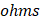# In order to quadruple the resistance of a uniform wire, a part of its length was uniformly stretched till the final length of the entire wire was 1.5 times the original length, the part of the wire was fraction equal toa)b)c)d)## Question ID - 150770 :- In order to quadruple the resistance of a uniform wire, a part of its length was uniformly stretched till the final length of the entire wire was 1.5 times the original length, the part of the wire was fraction equal toa)b)c)d)3537

(a)

Letbe the original length of wire andbe its length stretched uniformly such that final length isThenwhereororNext Question :
 The slope of plate characteristic of a vacuum tube diode for certain operating point on the curve is. The plate resistance of the diode and its nature respectively a) 100-static b) 1000-static c) 1000-dynamic d) 100-dynamic